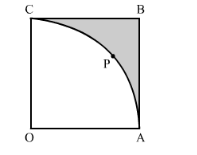# In the following figure, OABC is a square of side 7 cm.Question:

In the following figure, OABC is a square of side 7 cm. If OAPC is a quadrant of a circle with centre O, then find the area of the shaded region. (Use π = 22/7)Solution:

Area of shaded region = Area of square OABC − Area of quadrant OAPC

$=(\text { Side })^{2}-\frac{1}{4} \pi r^{2}$

$=(7)^{2}-\frac{1}{4} \times \frac{22}{7} \times 7 \times 7$

$=49-38.5$

$=10.5 \mathrm{~cm}^{2}$

Hence, the area of the shaded region is $10.5 \mathrm{~cm}^{2}$